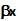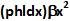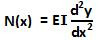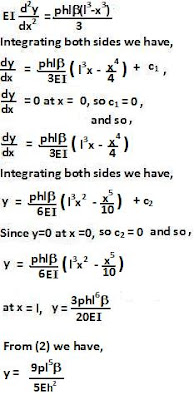## Monday, April 13, 2009

### Irodov Problem 1.304Consider an infinitesimally thin (of width dx) strip of the square plate at a distance x from the axis of rotation. These points are experiencing a linear tangential acceleration of. If the density of the plate is p and its thickness is h, then the mass of this infinitesimally think strip will be phldx. As seen from a frame that is rotating, this will translate to a pseudo force of magnitude. This pseudo force results in a pseudo torque of magnitudein a direction opposite to the direction of rotation of the plate.

Now let us consider the torques a section of the plate XB (as indicated in the figure). The net torque acting on this section of the plate due to the pseudo torque can be computed as,This pseudo torque will be exactly balanced by the torque due to elastic forces N(x) acting at the boundary of plate section at X. From Irodov Problem 1.301 (or this link) we know that the elastic curve of the plate is given by,Where, for this plate, as solved in Irodov Problem 1.303 part a,Hence, we have,